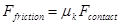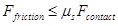# 02. Drawing Free-Body Diagrams

$$\newcommand{\vecs}{\overset { \rightharpoonup} {\mathbf{#1}} }$$ $$\newcommand{\vecd}{\overset{-\!-\!\rightharpoonup}{\vphantom{a}\smash {#1}}}$$$$\newcommand{\id}{\mathrm{id}}$$ $$\newcommand{\Span}{\mathrm{span}}$$ $$\newcommand{\kernel}{\mathrm{null}\,}$$ $$\newcommand{\range}{\mathrm{range}\,}$$ $$\newcommand{\RealPart}{\mathrm{Re}}$$ $$\newcommand{\ImaginaryPart}{\mathrm{Im}}$$ $$\newcommand{\Argument}{\mathrm{Arg}}$$ $$\newcommand{\norm}{\| #1 \|}$$ $$\newcommand{\inner}{\langle #1, #2 \rangle}$$ $$\newcommand{\Span}{\mathrm{span}}$$ $$\newcommand{\id}{\mathrm{id}}$$ $$\newcommand{\Span}{\mathrm{span}}$$ $$\newcommand{\kernel}{\mathrm{null}\,}$$ $$\newcommand{\range}{\mathrm{range}\,}$$ $$\newcommand{\RealPart}{\mathrm{Re}}$$ $$\newcommand{\ImaginaryPart}{\mathrm{Im}}$$ $$\newcommand{\Argument}{\mathrm{Arg}}$$ $$\newcommand{\norm}{\| #1 \|}$$ $$\newcommand{\inner}{\langle #1, #2 \rangle}$$ $$\newcommand{\Span}{\mathrm{span}}$$$$\newcommand{\AA}{\unicode[.8,0]{x212B}}$$

# Drawing Free-Body Diagrams

The free-body diagram is still the most important analysis tool for determining the forces that act on a particular object. As an example, start with a verbal description of a situation:

While rearranging furniture, a 600 N force is applied at an angle of 25°below horizontal to a 100 kg sofa at rest.

A free-body diagram for the sofa is sketched below:This is a complete free-body diagram for the couch.

The only non-contact interaction is the force of gravity, directed vertically downward.

The couch is in contact with two external objects, the person, pushing the couch across the floor, and the floor, exerting a force directed upward to prevent the couch from sinking into the floor. In addition, experience tells us that the interaction between the couch and the floor also hinders the motion of the couch in the direction of the person’s push. This portion of the couch-floor interaction is commonly referred to as friction.

# The Force of Friction

The interaction between objects in direct contact typically consists of two parts. One part of the interaction is directed perpendicular to the surface of contact.The other part of the interaction is the portion commonly called friction. The frictional portion of the interaction depends on many variables.

For most situations, a model of friction limiting the number of variables effecting the interaction to two is adequate. These two variables are the magnitude of the perpendicular portion of the interaction, generically called the contact force, and a unit-less constant that reflects the relative roughness of the surface-to-surface contact, termed the coefficient of friction. This linear model of sliding friction further differentiates between the frictional interaction when the two surfaces are moving with respect to each other, termed kinetic friction, and when they are not, termed static friction.

## Kinetic friction

The kinetic friction model states that the frictional interaction between the surfaces is approximately equal to the product of the contact force, Fcontactand the coefficient of friction for kinetic situations, mk;The direction of this force on a particular object is in opposition to the relative motion of the two surfaces in contact.

## Static friction

The static friction model states that the frictional interaction between the surfaces must be less than, or at most equal to, the product of the contact force, Fcontact, and the coefficient of friction for static situations, ms;The direction of this force on a particular object is in opposition to the motion that would result if the frictional interaction were not present.

The portion of the interaction directed perpendicular to the surface of contact is sometimes referred to as the normal force, where normal has its mathematical definition of perpendicular.

This page titled 02. Drawing Free-Body Diagrams is shared under a CC BY-NC-SA license and was authored, remixed, and/or curated by Paul D'Alessandris.